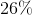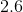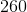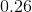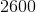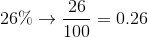# Algebra 1 : Decimals and Percentage

## Example Questions

### Example Question #4 : How To Find Decimal Equivalent To A Percentage

Fill in the missing information.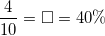Explanation:

When reading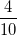aloud it readsout of, or "four tenths." This "four tenths" as a decimal is literally written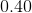We could also use the other term given to find our answer. Decimals are found from percentages by moving the decimal point two places to the left. Given, the decimal moved results in### Example Question #5 : How To Find Decimal Equivalent To A Percentage

Fill in the missing information.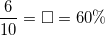Explanation:

When reading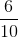aloud it readsout of, or "six tenths." This "six tenths" as a decimal is literally written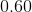.

We could also use the other term given to find our answer. Decimals are found from percentages by moving the decimal point two places to the left. Given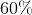, the decimal moved results in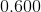or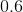### Example Question #1 : How To Find Decimal Equivalent To A Percentage

Solve the problem and convert the answer to a percentage.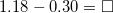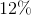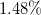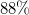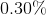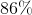Explanation: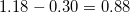. To convert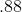to a percentage, the decimal is moved two places to the right, resulting in.

Alternatively, we would have converted the decimals to percetages from the beginnging.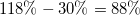, to do this we started with the decimal numbers given and moved the decimal point to the right two places.

### Example Question #1 : How To Find Decimal Equivalent To A Percentage

Convert this percentage to a decimal.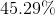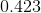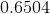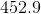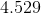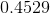Explanation:

Given the percentage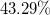, the decimal point must be moved two decimal places to the left, resulting in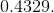This decimal can further be written as fraction,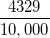which when reduced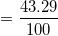.

Since percentages represent part of a whole, or part of 100,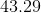is the final answer.

### Example Question #8 : How To Find Decimal Equivalent To A Percentage

Convert the answer to a decimal.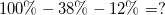Explanation:

First we must solve the problem: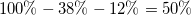.

Now to convert the percentage to a decimal all we do is move the decimal point two decimal places to the left. In this case, we began with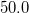. After moving the decimal point we end up with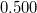as our result.

### Example Question #11 : How To Find Decimal Equivalent To A Percentage

Convert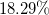to a decimal.Explanation:

To convert a percentage to a decimal, we simply move the decimal point two places to the left.

Alternatively, to convert from a decimal to a percentage, we would move the decimal place two decimal places the right.

But in this case, we are going from percentage to a decimal, so starting with, moving the decimal places two places to the left would leave us with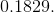Another way to solve is to divide the percentage by one hundred: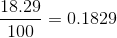This is our answer.

### Example Question #2981 : Algebra 1

Find the decimal equivalent of the following percent: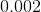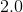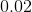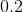Explanation:

In order to find the decimal equivalent for a percent, all we have to do is divide our percent by 100. We can also move the decimal point to the left two spaces.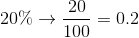### Example Question #13 : How To Find Decimal Equivalent To A Percentage

Find the decimal equivalent for the following percent: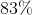Explanation:

In order to find the decimal equivalent for a percent, all we have to do is divide our percent by 100. We can also move the decimal point to the left two spaces.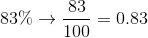### Example Question #14 : How To Find Decimal Equivalent To A Percentage

Find the decimal equivalent of the following percent: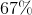Explanation:

In order to find the decimal equivalent for a percent, all we have to do is divide our percent by 100. We can also move the decimal point to the left two spaces.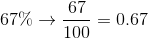### Example Question #51 : Decimals And Percentage

Find the decimal equivalent of the following percent: